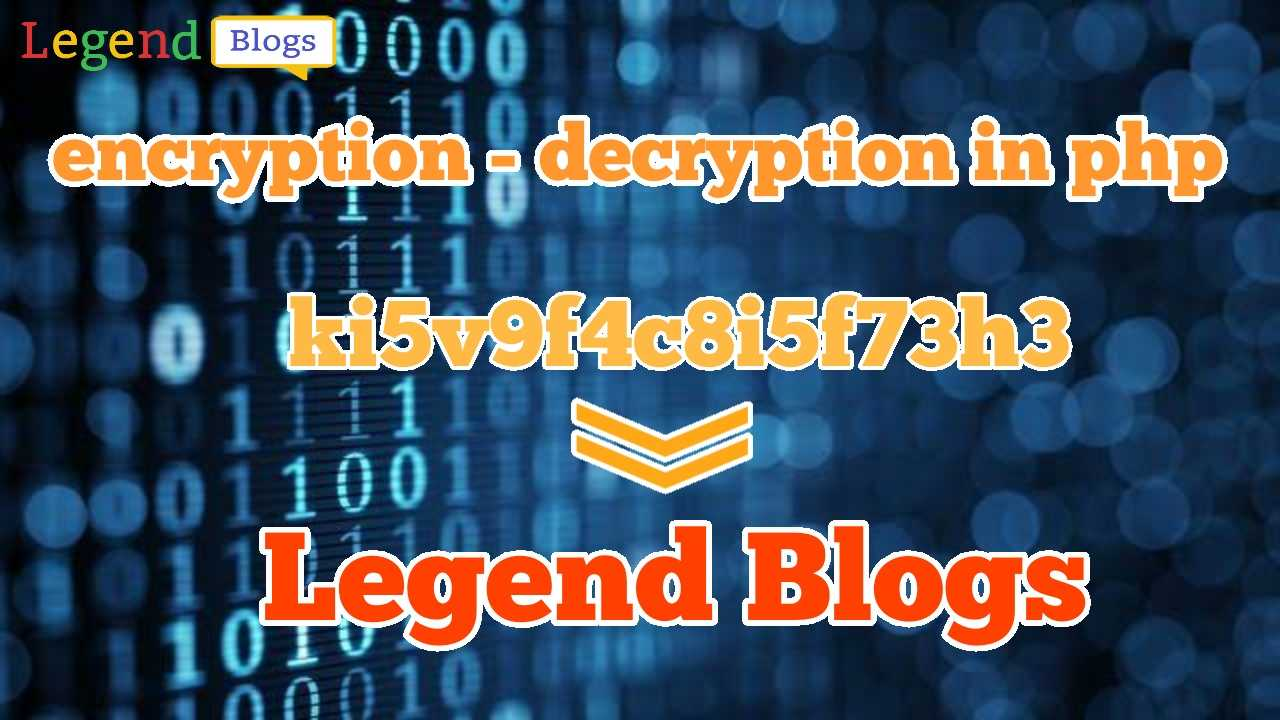## How do you Encrypt and Decrypt a PHP String## What is Mcrypt in PHP?

Mcrypt is a replacement for the popular Unix crypt command. the crypt was a file encryption tool that used an algorithm very close to the World War II Enigma cipher. Mcrypt provides the same functionality but uses several modern algorithms such as AES.

## What is encryption in PHP?

Algorithms that achieve one-way encryption are called hashing algorithms, and they work by taking a string (for example, IronMan) and then creating a unique fingerprint (if you like) from it. PHP offers a hashing algorithm called MD5, which basically takes a string and returns a 128-bit fingerprint of it.

## What is md5 in PHP?

The md5() function uses the RSA Data Security, Inc. MD5 Message-Digest Algorithm. From RFC 1321 - The MD5 Message-Digest Algorithm: "The MD5 message-digest algorithm takes as input a message of arbitrary length and produces as output a 128-bit "fingerprint" or "message digest" of the input.

## Let's do it with Mcrypt:

First of all, create a script for encrypting:

``````function encrypt(\$str, \$isBinary = false) {
\$iv = \$this->iv;
\$str = \$isBinary ? \$str : utf8_decode(\$str);
\$td = mcrypt_module_open('rijndael-128', ' ', 'cbc', \$iv);
mcrypt_generic_init(\$td, \$this->key, \$iv);
\$encrypted = mcrypt_generic(\$td, \$str);
mcrypt_generic_deinit(\$td);
mcrypt_module_close(\$td);
return \$isBinary ? \$encrypted : bin2hex(\$encrypted);
}``````

After that, create a script for decrypting:

``````function decrypt(\$code, \$isBinary = false) {
\$code = \$isBinary ? \$code : \$this->hex2bin(\$code);
\$iv = \$this->iv;
\$td = mcrypt_module_open('rijndael-128', ' ', 'cbc', \$iv);
mcrypt_generic_init(\$td, \$this->key, \$iv);
\$decrypted = mdecrypt_generic(\$td, \$code);
mcrypt_generic_deinit(\$td);
mcrypt_module_close(\$td);
return \$isBinary ? trim(\$decrypted) : utf8_encode(trim(\$decrypted));
}``````

In both functions a function is called hex2bin(), so now just create it:

``````function hex2bin(\$hexdata) {
\$bindata = '';
for (\$i = 0; \$i < strlen(\$hexdata); \$i += 2) {
\$bindata .= chr(hexdec(substr(\$hexdata, \$i, 2)));
}
return \$bindata;
}``````

Now, combine all of them into a class file:

``````class MCrypt {

private \$iv = 'abcdef9876541720';
private \$key = '1720456789fedcba';

function __construct() {

}

/**
* @param string \$str
* @param bool \$isBinary whether to encrypt as binary or not. Default is: false
* @return string Encrypted data
*/
function encrypt(\$str, \$isBinary = false) {
\$iv = \$this->iv;
\$str = \$isBinary ? \$str : utf8_decode(\$str);
\$td = mcrypt_module_open('rijndael-128', ' ', 'cbc', \$iv);
mcrypt_generic_init(\$td, \$this->key, \$iv);
\$encrypted = mcrypt_generic(\$td, \$str);
mcrypt_generic_deinit(\$td);
mcrypt_module_close(\$td);
return \$isBinary ? \$encrypted : bin2hex(\$encrypted);
}

/**
* @param string \$code
* @param bool \$isBinary whether to decrypt as binary or not. Default is: false
* @return string Decrypted data
*/
function decrypt(\$code, \$isBinary = false) {
\$code = \$isBinary ? \$code : \$this->hex2bin(\$code);
\$iv = \$this->iv;
\$td = mcrypt_module_open('rijndael-128', ' ', 'cbc', \$iv);
mcrypt_generic_init(\$td, \$this->key, \$iv);
\$decrypted = mdecrypt_generic(\$td, \$code);
mcrypt_generic_deinit(\$td);
mcrypt_module_close(\$td);
return \$isBinary ? trim(\$decrypted) : utf8_encode(trim(\$decrypted));
}

protected function hex2bin(\$hexdata) {
\$bindata = '';
for (\$i = 0; \$i < strlen(\$hexdata); \$i += 2) {
\$bindata .= chr(hexdec(substr(\$hexdata, \$i, 2)));
}
return \$bindata;
}

}``````

Well, now create an object of your class and use it:

``````function encryptkey(\$key) {
\$mcrypt = new MCrypt();
\$encrypted = \$mcrypt->encrypt(\$key);
return \$encrypted;
}

function decryptkey(\$key) {
\$mcrypt = new MCrypt();
\$decrypted = \$mcrypt->decrypt(\$key);
return \$decrypted;
}

\$value = "Legend Blogs";

echo \$value.'<br>';
echo "Encrypt: ". encryptkey(\$value).'<br>';
echo "Decrypt: ". decryptkey(encryptkey(\$value)).'<br>';``````

## Output:

``````Legend Blogs
Encrypt: 651e88099b127fc1599349228426a858
Decrypt: Legend Blogs``````